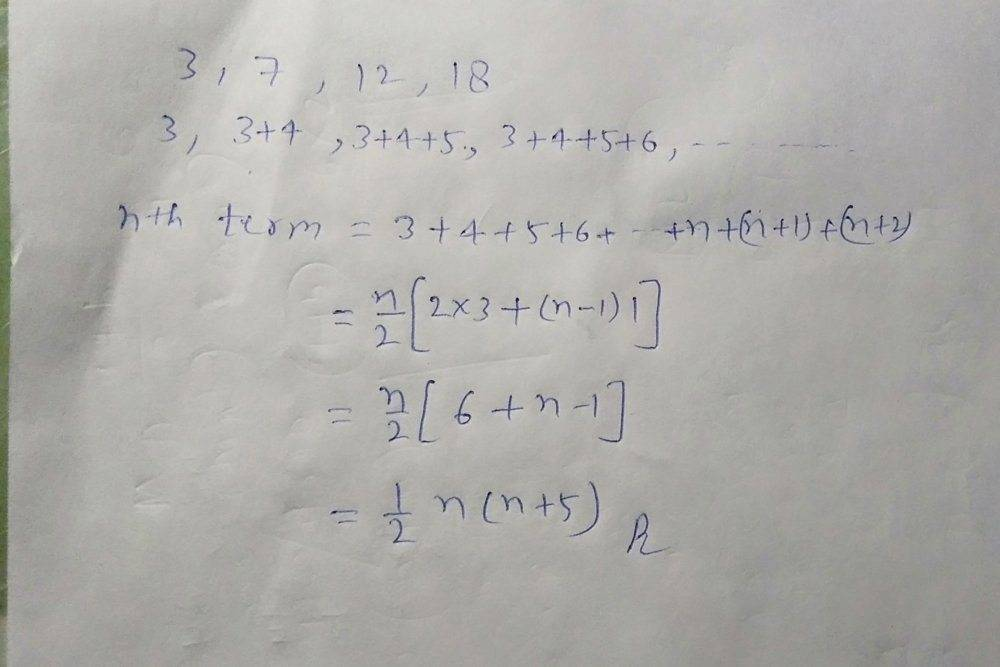# Solve 3 7 12 18 25 series

• B
• johann1301h

#### johann1301h

Hi, I am trying to solve this series generally:

the series: 3 7 12 18 25.

i tried using x(n) = 3 + 4n.

Hi, I am trying to solve this series generally:

the series: 3 7 12 18 25.

i tried using x(n) = 3 + 4n.

I would add ##0## at the beginning and see whether that helps.

Calculate how much the numbers increase in each step. That should lead to some pattern.

Hi, I am trying to solve this series generally:

the series: 3 7 12 18 25.

i tried using x(n) = 3 + 4n.

Did you try graphing it?
When I graphed your series of numbers, I got a curve.
That implied to me, that "4n", being a linear function, was not correct.

You must find ##f(n)## such that ##a_{n+1}=f(n)+a_{n}## with ##a_{0}=3##, hint: think simple!
Ssnow

•Aufbauwerk 2045
Most sequences can be resolve using difference (not differential) analysis.

Code:
 3
7    4
12    5    1
18    6    1
25    7    1

•Aufbauwerk 2045
Just stumbled across this page. I'm doing independent math research and the series you are inquiring about is a smaller part of what I am doing. The formula you are looking for is F(n)= n(n+5) / 2 = {3,7,12,18,25,...}. It is similar to the formula for triangular numbers T(n) = n(n+1)/2 = {1,3,6,10,15,...}. Hope this helps!

Regards,
David

Difference between two numbers is (2+n), so you can write x(n)= (2+n) + x(n-1) when n > 1.

This is a recursive formula, that also can be written out as:
x(n) = (2+n) + (2+n-1) + (2+n-2) + (2+n-3) + ... + x(1)

Recognizing the pattern as:
$x(n) = x(1) + \sum_{i=2}^n(2+i) = x(1) - 3 + \sum_{i=1}^n(2+i) = \sum_{i=1}^n2+ \sum_{i=1}^ni = 2n+ n(n+1)/2 = n(n+5)/2$

So we find x(n) = n(n+5)/2.

$\sum_{i=1}^nn = n(n+1)/2$ can be found from a table or WolframAlpha(or could also derivated without too much trouble) :)

Type out math instead of posting images.This is not necessarily a better method, since yours is actually probably faster in this case, however any series with a constant second difference is called a quadratic series and can be generalised as such:

##u_{n} = an^{2} + bn + c##

First term: ##u_{1} = a + b + c##
First difference: ##u_{n} - u_{n-1} = 2an + (b-a)##
Second difference: ##(u_{n} - u_{n-1}) - (u_{n-1} - u_{n-2}) = 2a##

So in the case of the sequence ##3,7,12,18,25...## we can write down three relations

##a + b + c = 3##
##3a + b = 4##
##2a = 1##

Which gives ##a = \frac{1}{2}, b = \frac{5}{2}, c= 0##, so

##u_{n} = \frac{1}{2}n^{2} + \frac{5}{2}n##

which is the answer you obtained.

More generally it's easy to check by induction that $$\sum_{n=1}^{a} n (n + 1) (n + 2) \dotsm (n + p - 1) = \frac{a (a + 1) \dotsm (a + p)}{p + 1} \,.$$ The relation can be be seen as the discrete analogue of $$\int_{0}^{a} \mathrm{d}x \, x^{p} = \frac{a^{p+1}}{p + 1}$$ and it can be used to determine the partial sum of any polynomial. For example, $$\begin{eqnarray*} \sum_{n=1}^{a} n^{3} &=& \sum_{n=1}^{a} \bigl( n - 3 n (n + 1) + n (n + 1) (n + 2) \bigr) \\ &=& \frac{1}{2} a (a + 1) - 3 \frac{1}{3} a (a + 1) (a + 2) + \frac{1}{4} a (a + 1) (a + 2) (a + 3) \\ &=&\frac{1}{4} a^{2} (a + 1)^{2} \,. \end{eqnarray*}$$